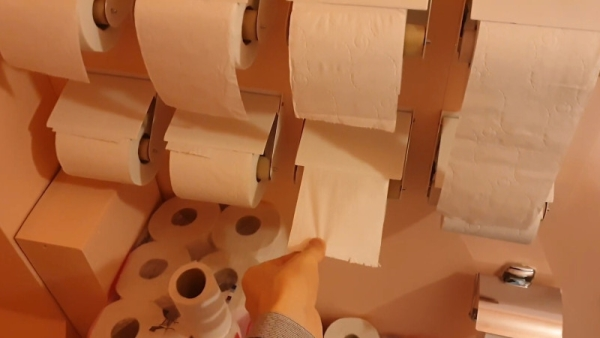# Weather-station With Arduino, BME280 & Display for Seeing the Trend Within the Last 1-2 Days

Here on instructables weather stations have already been introduced. They show the current air pressure, temperature and humidity. What they lacked so far was a presentation of the course within the last 1-2 days. This process would have the advantage that you can not only graphically read the current values but also at a glance, see how they have changed in the last 1-2 days. As a result, one recognizes, for example, a possible change in weather, as the air pressure changes greatly. However, one also recognizes general relationships between the measured quantities.

For example, the humidity decreases when the air temperature rises. This is because warm air can absorb more humidity than cold air. If the relative humidity is about 60% at 20 ° C, then at 25 ° C the air could absorb more humidity in absolute terms. Therefore, the relative humidity is no longer 60%, but for example, only 50% off.

Also you can see nicely what time of day the highest or lowest temperatures are to be expected. Or that the humidity rises sharply when it rains. Ideal for the hobby meteorologist. I’d be very happy if you could post your experiences in the comments.

## Step 1: Parts

For this weather station you only need 5 parts:

* Arduino mega: ebay arduino mega

* Weather sensor BME280: ebay BME280

* 320×480 pixel display for the Arduino Mega: ebay 320×480 display

* + 9V power supply: ebay power supply

* Electric wire

The total costs are only less than \$25.

## Step 2: The Arduino Code

The circuit is very simple. You just have to connect the sensor to the arduino mega at this way:

Vin –> +5V

GND –> GND

SDA –> pin 20

SCL –> pin 21

The display is only plugged into the connector strip on the arduino mega.

Here are the links for the arduino-libraries you’ll need:

The heart of this weather station is, as I said, the graphical representation of the weather data. At the moment, the values are updated every 6 minutes and the graphs are shifted 1 pixel to the left. In this way, the last 1.5 days can be recorded. Of course this can be changed at any time. Only then the value 360000 ms (= 6 minutes) and of course the time axis in hours must be changed. Here are the lines you’ll have to change:

time_neu = millis();

if(time_neu < time_alt) // to avoid problems after the millis-overflow

{

time_next = 0 + 360000;

}

if(time_neu > time_next && time_next >= 360000) // new measurement after 6 minutes

{

I have decided to keep the temperature, air pressure and humidity scales unchanged, as it allows you to quickly assess, over time, whether the air pressure is high, medium or low, based on the location of the current readings. If I would adjust the scale again and again, I would not recognize this at first glance. The time axis is located at the position y = 290 pixels. The marks on the y-axes are 45 pixels apart. If you want to display the air pressure from 940 mbar to 1000 mbar in 10 mbar steps, proceed as follows:

First, set up the general equation y = k * x + d. Now you use those 2 value pairs (x = 940, y = 290) and (x = 950, y = 245). This gives 2 equations with the two unknowns k and d: 290 = k * 940 + d and 245 = k * 950 + d. By subtracting both equations, we get: 290 – 245 = k * 940 – k * 950 + d – d. The unknown d vanishes in this way and we obtain for k = – 45/10 = -4.5. This value for k is placed in one of the two initial equations: 290 = -4.5 * 940 + d. In this way one obtains the value for d, specifically d = 4520.

If you want the air pressure, for example represent only 955 mbar to 985 mbar, you put the value pairs (955, 290) and (960, 245) in the straight-line equation. Then one obtains for k = -9 and d = 8885. Similarly, one calculates the straight-line equations for the temperature and the air humidity. These 3 equations appear here in the program:

for (i = 0; i <= 348; i ++)

{

if (humidity [i]! = -66)

{

myGLCD.setColor (255,0,0);

//myGLCD.drawPixel(81 + i, -4.5 * temperature [i] + 200);

myGLCD.drawLine (81 + i, -4.5 * temperature [i] + 200.81 + i + 1, -4.5 * temperature [i + 1] + 200);

myGLCD.setColor (0,255,0);

//myGLCD.drawPixel(81 + i, -4.5 * humidity [i] + 380);

myGLCD.drawLine (81 + i, -4.5 * humidity [i] + 380.81 + i + 1, -4.5 * humidity [i + 1] + 380);

myGLCD.setColor (0,0,255);

//myGLCD.drawPixel(81 + i, -4.5 * pressure [i] + 4520);

myGLCD.drawLine (81 + i, -9.0 * pressure [i] + 8885,81 + i + 1, -9.0 * pressure [i + 1] + 8885);

}

}

## Step 3: The Results

One word to the video: To make the expansion of the graph visible I decreased the time-steps down to 1 second. Therefore the display is strongly flickering. In reality the time-steps are 6 minutes. So you can’t see any flickering…

I would be glad if one or the other hobby meteorologist tries to tinker my weather station. A comparison with official measuring stations (f.e. University of Graz/austria) shows the usability of the measuring curves.

Furthermore, I would be happy if you could vote for me in the sensor contest and for my other instructables in the classroom science contest:

Thanks a lot for this.

If you are interested in more physics projects, here is my youtube channel: https://www.youtube.com/user/stopperl16/videos

In this sense, Eureka …